# A.1 Convexity of Sets and Domains

A subset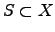is defined to be convex if for arbitrarily chosen points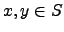the connection line between these two points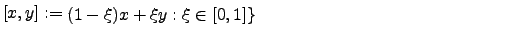(A.1)

is completely included in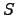[219,348]. Therefore, the implication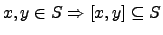(A.2)

can be applied. Figure A.1 shows two typical parameter domains. The left domain in this figure is convex but the second one has obviously not a convex nature.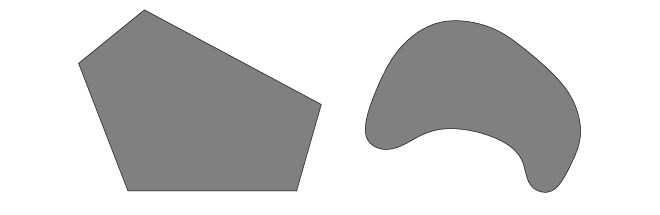For unconstraint parameters of an optimization problem the parameter domain is convex. If non-linear constraint functions are applied to this problem the parameter space might be become non-convex. If penalty or barrier functions are used instead of constraint functions the objective function becomes more complex but the lemmas for the convergence of these optimization problems can be again applied as for unconstraint optimization [219,220].

Stefan Holzer 2007-11-19